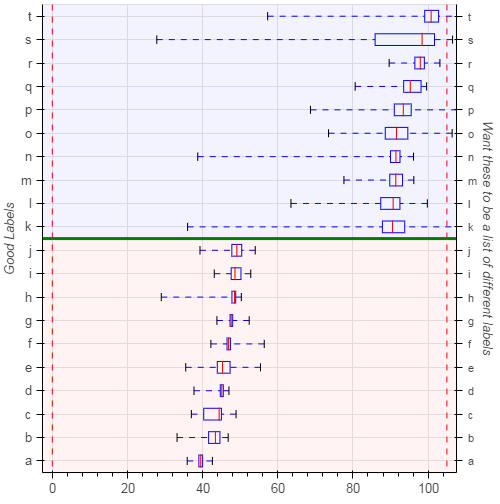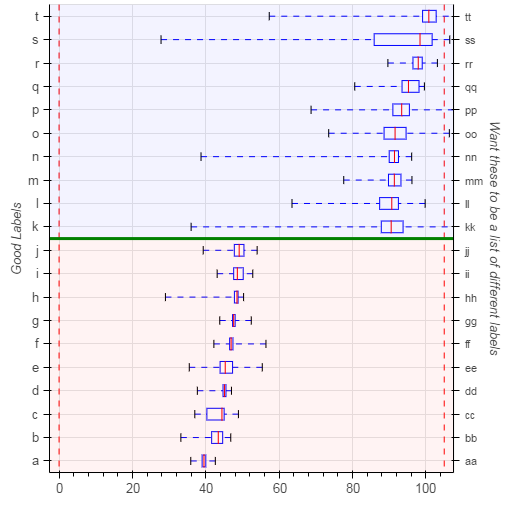# Categorical extra_y_range issue (code included)

All,
I am having an issue with trying to apply a text label to the right axis that aligns with the horizontal boxes. I need to add an additional label to the right in text with a further description of the box details.

I would like help in trying to get the “right_axis_labels” on the right axis. I have no issue with the numerical axis but for this the picture is as close as I have been able to get.

I have provided all code minus the box plot data because I am still new to python and am not sure how to efficiently generate fake data. I would also appreciate any tips you may have on improving the code.

import pandas as pd

from bokeh.plotting import figure, show

from bokeh.models import plots, Span, BoxAnnotation, Range1d, CategoricalAxis

cats = [‘a’, ‘b’, ‘c’, ‘d’, ‘e’, ‘f’, ‘g’, ‘h’, ‘i’, ‘j’, ‘k’, ‘l’, ‘m’, ‘n’, ‘o’, ‘p’, ‘q’, ‘r’, ‘s’, ‘t’]

# inputs

units = ‘defF’

lim_min = 0

lim_max = 105

space = (lim_max - lim_min) * 0.025

val_max = df[‘max’]

val_min = df[‘min’]

q1 = df[‘q1’]

med = df[‘med’]

q3 = df[‘q3’]

customer = df[‘customer’].values

p = figure(width=500, height=500, y_range=cats, x_range=Range1d(lim_min-space, lim_max+space))

# p.xaxis.axis_label = ‘Units: %s’ %units

p.xaxis.axis_label_text_font_size = ‘10pt’

p.xaxis.major_label_text_font_size = ‘10pt’

p.yaxis.major_label_text_font_size = ‘10pt’

p.yaxis.axis_label = ‘Good Labels’

p.yaxis.axis_label_text_font_size = ‘10pt’

p.toolbar_location = None

box_height = .5

# wiskers

p.segment(val_max, cats, q3, cats, line_dash=‘dashed’, line_color=“blue”)

p.segment(val_min, cats, q1, cats, line_dash=‘dashed’, line_color=“blue”)

# boxes

p.hbar(cats, box_height, q1, q3, fill_color=“white”, line_color=“blue”)

# caps (almost-0 height rects simpler than segments)

p.rect(val_min, cats, 0.01, box_height*0.6, line_color=“black”)

p.rect(val_max, cats, 0.01, box_height*0.6, line_color=“black”)

# median line

p.rect(med, cats, 0.01, box_height, line_width=1, line_color=“red”)

# limit lines

limit_min_line = Span(location=lim_min,

dimension=‘height’, line_color=‘red’,

line_dash=‘dashed’, line_width=1)

limit_max_line = Span(location=lim_max,

dimension=‘height’, line_color=‘red’,

line_dash=‘dashed’, line_width=1)

# add top and bottom areas

low_box = BoxAnnotation(top=split+.5, fill_alpha=0.05, fill_color=‘red’)

high_box = BoxAnnotation(bottom=split+.5, fill_alpha=0.05, fill_color=‘blue’)

top_2_bot_split = Span(location=split+.5, dimension=‘width’, line_color=‘green’, line_width=3)

p.extra_y_ranges={‘right’:p.y_range}

p.add_layout(CategoricalAxis(y_range_name=‘right’, axis_label=“Want these to be a list of different labels”), ‘right’)

right_axis_labels = [‘aa’, ‘bb’, ‘cc’, ‘dd’, ‘ee’, ‘ff’ ,‘gg’, ‘hh’, ‘ii’, ‘jj’, ‘kk’, ‘ll’, ‘mm’, ‘nn’, ‘oo’, ‘pp’, ‘qq’, ‘rr’, ‘ss’, ‘tt’]

show§Hi,

Categorical axes just display the categories in their associated range. You are setting your extra range to be the same range as the right side (the default range), so I would expect the labels to be the same. If you want different labels you could try:

p.extra_y_ranges={ 'right': FactorRange(*right_axis_labels) }

But I can't say that I've ever tried multiple axes with different factor ranges (only numerical ones) so I can't guarantee this will work.

Other options include not adding an extra range at all, but simply using a FuncTickFormatter to format the existing factors however you want:

right_axis = CategoricalAxis()
right_axis.formatter = FuncTickFormatter(code="""
# JS code to map "a" to description for "a", etc
""")

http://bokeh.pydata.org/en/latest/docs/user_guide/styling.html#functickformatter

Lastly, a PR was just merged that would let you easily set "label overrides" directly on an axis:

But note that this is only available on master/dev builds currently, it is not yet in any stable release (it will be in 0.12.6 later this month).

Thanks,

Bryan

···

On May 12, 2017, at 17:59, David G <[email protected]> wrote:

All,
I am having an issue with trying to apply a text label to the right axis that aligns with the horizontal boxes. I need to add an additional label to the right in text with a further description of the box details.

I would like help in trying to get the "right_axis_labels" on the right axis. I have no issue with the numerical axis but for this the picture is as close as I have been able to get.

I have provided all code minus the box plot data because I am still new to python and am not sure how to efficiently generate fake data. I would also appreciate any tips you may have on improving the code.

import pandas as pd
from bokeh.plotting import figure, show
from bokeh.models import plots, Span, BoxAnnotation, Range1d, CategoricalAxis
cats = ['a', 'b', 'c', 'd', 'e', 'f', 'g', 'h', 'i', 'j', 'k', 'l', 'm', 'n', 'o', 'p', 'q', 'r', 's', 't']

# plotting
# inputs
units = 'defF'
lim_min = 0
lim_max = 105
space = (lim_max - lim_min) * 0.025

val_max = df['max']
val_min = df['min']
q1 = df['q1']
med = df['med']
q3 = df['q3']
customer = df['customer'].values

p = figure(width=500, height=500, y_range=cats, x_range=Range1d(lim_min-space, lim_max+space))

# p.xaxis.axis_label = 'Units: %s' %units
p.xaxis.axis_label_text_font_size = '10pt'
p.xaxis.major_label_text_font_size = '10pt'

p.yaxis.major_label_text_font_size = '10pt'
p.yaxis.axis_label = 'Good Labels'

p.yaxis.axis_label_text_font_size = '10pt'
p.toolbar_location = None

box_height = .5

# wiskers
p.segment(val_max, cats, q3, cats, line_dash='dashed', line_color="blue")
p.segment(val_min, cats, q1, cats, line_dash='dashed', line_color="blue")

# boxes
p.hbar(cats, box_height, q1, q3, fill_color="white", line_color="blue")

# caps (almost-0 height rects simpler than segments)
p.rect(val_min, cats, 0.01, box_height*0.6, line_color="black")
p.rect(val_max, cats, 0.01, box_height*0.6, line_color="black")

# median line
p.rect(med, cats, 0.01, box_height, line_width=1, line_color="red")

# limit lines
limit_min_line = Span(location=lim_min,
dimension='height', line_color='red',
line_dash='dashed', line_width=1)
limit_max_line = Span(location=lim_max,
dimension='height', line_color='red',
line_dash='dashed', line_width=1)

# add top and bottom areas
low_box = BoxAnnotation(top=split+.5, fill_alpha=0.05, fill_color='red')
high_box = BoxAnnotation(bottom=split+.5, fill_alpha=0.05, fill_color='blue')

top_2_bot_split = Span(location=split+.5, dimension='width', line_color='green', line_width=3)

p.extra_y_ranges={'right':p.y_range}
p.add_layout(CategoricalAxis(y_range_name='right', axis_label="Want these to be a list of different labels"), 'right')

right_axis_labels = ['aa', 'bb', 'cc', 'dd', 'ee', 'ff' ,'gg', 'hh', 'ii', 'jj', 'kk', 'll', 'mm', 'nn', 'oo', 'pp', 'qq', 'rr', 'ss', 'tt']

show(p)

--
You received this message because you are subscribed to the Google Groups "Bokeh Discussion - Public" group.
To unsubscribe from this group and stop receiving emails from it, send an email to [email protected].
To post to this group, send email to [email protected].
To view this discussion on the web visit https://groups.google.com/a/continuum.io/d/msgid/bokeh/00212c3d-f169-4530-9d79-c897f95cfa3d%40continuum.io.
<bokeh hboxplot.png>

Thanks Bryan. That works perfectly.

Revised code portion for future reference.

right_axis_labels = [‘aa’, ‘bb’, ‘cc’, ‘dd’, ‘ee’, ‘ff’ ,‘gg’, ‘hh’, ‘ii’, ‘jj’, ‘kk’, ‘ll’, ‘mm’, ‘nn’, ‘oo’, ‘pp’, ‘qq’, ‘rr’, ‘ss’, ‘tt’]

p.extra_y_ranges={‘right’:FactorRange(*right_axis_labels)}

p.add_layout(CategoricalAxis(y_range_name=‘right’, axis_label=“Want these to be a list of different labels”), ‘right’)

``···

On Saturday, May 13, 2017 at 8:33:34 AM UTC-5, Bryan Van de ven wrote:

Hi,

Categorical axes just display the categories in their associated range. You are setting your extra range to be the same range as the right side (the default range), so I would expect the labels to be the same. If you want different labels you could try:

``````    p.extra_y_ranges={ 'right': FactorRange(*right_axis_labels) }
``````

But I can’t say that I’ve ever tried multiple axes with different factor ranges (only numerical ones) so I can’t guarantee this will work.

Other options include not adding an extra range at all, but simply using a FuncTickFormatter to format the existing factors however you want:

``````    right_axis = CategoricalAxis()

right_axis.formatter = FuncTickFormatter(code="""

# JS code to map "a" to description for "a", etc

""")

``````

``````    [http://bokeh.pydata.org/en/latest/docs/user_guide/styling.html#functickformatter](http://bokeh.pydata.org/en/latest/docs/user_guide/styling.html#functickformatter)
``````

Lastly, a PR was just merged that would let you easily set “label overrides” directly on an axis:

``````    [https://github.com/bokeh/bokeh/pull/6225](https://github.com/bokeh/bokeh/pull/6225)
``````

But note that this is only available on master/dev builds currently, it is not yet in any stable release (it will be in 0.12.6 later this month).

Thanks,

Bryan

On May 12, 2017, at 17:59, David G [email protected] wrote:

All,

I am having an issue with trying to apply a text label to the right axis that aligns with the horizontal boxes. I need to add an additional label to the right in text with a further description of the box details.

I would like help in trying to get the “right_axis_labels” on the right axis. I have no issue with the numerical axis but for this the picture is as close as I have been able to get.

I have provided all code minus the box plot data because I am still new to python and am not sure how to efficiently generate fake data. I would also appreciate any tips you may have on improving the code.

import pandas as pd

from bokeh.plotting import figure, show

from bokeh.models import plots, Span, BoxAnnotation, Range1d, CategoricalAxis

cats = [‘a’, ‘b’, ‘c’, ‘d’, ‘e’, ‘f’, ‘g’, ‘h’, ‘i’, ‘j’, ‘k’, ‘l’, ‘m’, ‘n’, ‘o’, ‘p’, ‘q’, ‘r’, ‘s’, ‘t’]

# inputs

units = ‘defF’

lim_min = 0

lim_max = 105

space = (lim_max - lim_min) * 0.025

val_max = df[‘max’]

val_min = df[‘min’]

q1 = df[‘q1’]

med = df[‘med’]

q3 = df[‘q3’]

customer = df[‘customer’].values

p = figure(width=500, height=500, y_range=cats, x_range=Range1d(lim_min-space, lim_max+space))

# p.xaxis.axis_label = ‘Units: %s’ %units

p.xaxis.axis_label_text_font_size = ‘10pt’

p.xaxis.major_label_text_font_size = ‘10pt’

p.yaxis.major_label_text_font_size = ‘10pt’

p.yaxis.axis_label = ‘Good Labels’

p.yaxis.axis_label_text_font_size = ‘10pt’

p.toolbar_location = None

box_height = .5

# wiskers

p.segment(val_max, cats, q3, cats, line_dash=‘dashed’, line_color=“blue”)

p.segment(val_min, cats, q1, cats, line_dash=‘dashed’, line_color=“blue”)

# boxes

p.hbar(cats, box_height, q1, q3, fill_color=“white”, line_color=“blue”)

# caps (almost-0 height rects simpler than segments)

p.rect(val_min, cats, 0.01, box_height*0.6, line_color=“black”)

p.rect(val_max, cats, 0.01, box_height*0.6, line_color=“black”)

# median line

p.rect(med, cats, 0.01, box_height, line_width=1, line_color=“red”)

# limit lines

limit_min_line = Span(location=lim_min,

``````                  dimension='height', line_color='red',
``````
``````                  line_dash='dashed', line_width=1)
``````

limit_max_line = Span(location=lim_max,

``````                  dimension='height', line_color='red',
``````
``````                  line_dash='dashed', line_width=1)
``````

# add top and bottom areas

low_box = BoxAnnotation(top=split+.5, fill_alpha=0.05, fill_color=‘red’)

high_box = BoxAnnotation(bottom=split+.5, fill_alpha=0.05, fill_color=‘blue’)

top_2_bot_split = Span(location=split+.5, dimension=‘width’, line_color=‘green’, line_width=3)

p.extra_y_ranges={‘right’:p.y_range}

p.add_layout(CategoricalAxis(y_range_name=‘right’, axis_label=“Want these to be a list of different labels”), ‘right’)

right_axis_labels = [‘aa’, ‘bb’, ‘cc’, ‘dd’, ‘ee’, ‘ff’ ,‘gg’, ‘hh’, ‘ii’, ‘jj’, ‘kk’, ‘ll’, ‘mm’, ‘nn’, ‘oo’, ‘pp’, ‘qq’, ‘rr’, ‘ss’, ‘tt’]

show§

You received this message because you are subscribed to the Google Groups “Bokeh Discussion - Public” group.

To unsubscribe from this group and stop receiving emails from it, send an email to [email protected].

To post to this group, send email to [email protected].

To view this discussion on the web visit https://groups.google.com/a/continuum.io/d/msgid/bokeh/00212c3d-f169-4530-9d79-c897f95cfa3d%40continuum.io.